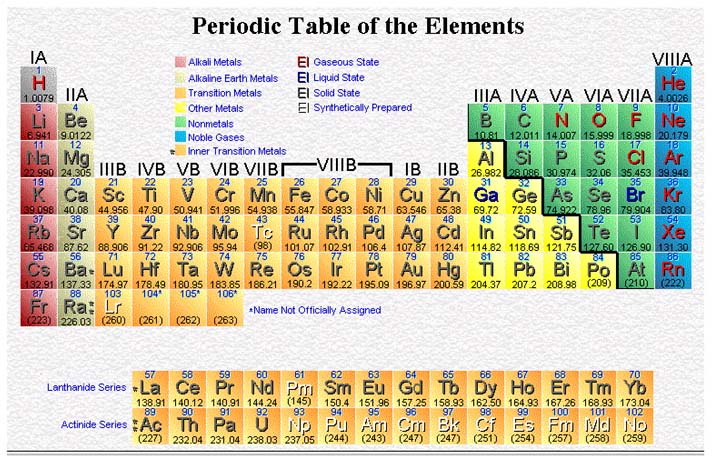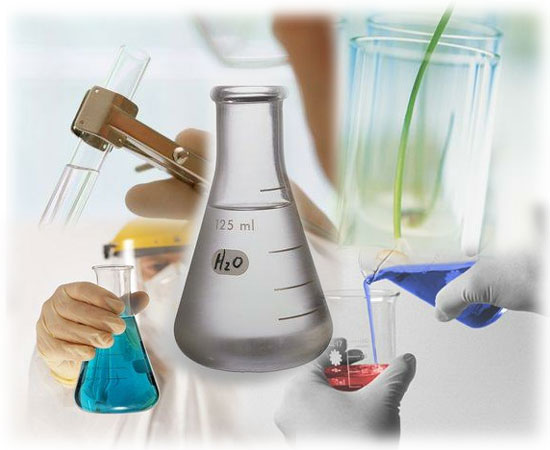CHEMISTRY Pre-AP Unit 8: The Mole Concept Chapter 10Goal: To develop the concept of the mole as a useful measurement and to apply this in calculations involving mass and volume.

Objectives: The student should be able to:

1. Define the mole in terms of Avogadro's number, formula mass or molecular mass.

2. Given the number of moles, determine the number of molecules of a covalent compound, formula units of an ionic compound or atoms of an element.

3. Given the number of particles present in a sample, determine the number of moles.

4. When given the formula, calculate the molecular mass of a covalent compound or the formula mass of an ionic compound.

5. Given the number of moles in a substance, determine the mass of the substance.

6. Given the mass of a substance, determine the number of moles of the substance.

7. When given the formula, calculate the percentage composition of each of the elements in the compound.

8. Given masses of the constituents or percentage composition data, determine the empirical formula of a compound.

9. Determine the molecular formula when the empirical formula is given or can be calculated and the molecular mass is given.

10. When given the formula or experimental data, calculate the percentage water in a hydrated compound.

11. Determine the empirical formula of a hydrated compound when given the percentage composition or the masses of the anhydrous substance and the water present.

Activities:

Nov 20: Discuss Unit VII Test, Lecture The Mole, Molar Mass

Nov 21: Discuss Practice Problems, Lecture Moles of Compounds and Percent Composition

Nov 27: Discuss Practice Problems, Begin Lecture Empirical and Molecular Formulas

Nov 28: Quiz: Mole Conversions, Finish Lecture Empirical and Molecular Formulas

Nov 29: Discuss Practice Problems, Lecture The Formula of a Hydrate

Nov 30: Lab: Formula of a Hydrate

Dec 1: Discuss Practice Problems; Ch 11 Assessment

Dec 4: Review for test

Dec 5: Unit VIII Test

Homework:

Nov 20: Practice Problems p311, 312, 316, 318

Nov 21: Practice Problems p321, 322, 323, 324, 326, 331, Mole Poster due Nov 29

Nov 27: Study for Quiz

Nov 28: Practice Problems p333, 335, 337

Nov 29: Practice Problems p340, Prelab for Hydrated Crystals

Dec 1: Lab Report Due Dec 6, Chapter 11 Assessment p346-349

Dec 4: Study for Unit VIII Test

Dec 5: Read Chapter 12, Work on lab report.

Lecture & Lab Materials (uploaded once per week):